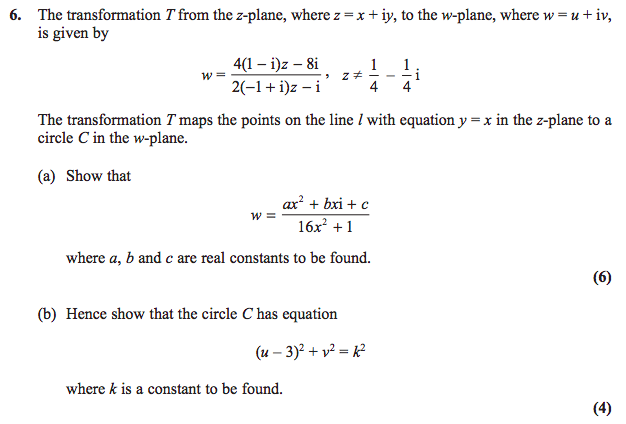You know how I often bang on about how ‘impossible’ exams are really nothing of the sort? Well, just for a change, I’m going to bang on about how sometimes exam boards get it wrong.

I’m looking at the 2014 Edexcel FP2 paper (the normal one, not the (R) one or the IAL one), in which this appears:Have a go at it before reading on, if you want to. Below the line be spoilers.

I’ll concede, happily, that I didn’t get the answer to part (b) within a reasonable time in class ((Chalk one up for ‘modelling resilience’)), so I enlisted some help.

Part (a), on the other hand, falls apart quite easily if you’re careful with your signs: in the end, $w = \frac{-32x^2 + 40x i + 8}{16x^2+1}$.

### @dragon_dodo and @realityminus3’s approach

I invited two of my good friends to have a stab at this, and they solved it with essentially the same approach: eliminate $x$ - a trick I completely missed.

We have $u = \frac{8 - 32x^2}{16x^2+1}$ and $v = \frac{40x}{16x^2+1}$. Rearranging the first equation gives $(16x^2+1) u = 8 - 32x^2$, or $x^2(16u+32) = 8 - u$, making $16x^2 = \frac{8-u}{u+2}$. (There are a lot of 16s around, and this will save a bit of expanding and factorising later.)

We then have $v^2 = \frac{1600x^2}{(16x^2+1)^2}$.

Now, $16x^2 + 1 = \frac{8-u}{u+2} + 1 = \frac{ 10}{u+2}$, so $(16x^2+1)^2 = \frac{100}{(u+2)^2}$.

This makes $v^2 = \frac{ 100 \frac{8-u}{u+2}}{\frac{100}{(u+2)^2}}$; multiplying top and bottom by $\frac{(u+2)^2}{100}$ makes the whole thing $v^2 - (8-u)(u+2)=0$.

Expanding, $v^2 - (16 +6u - u^2)=0$, or $u^2 - 6u + v^2 = 16$; that can be written as $(u-3)^2 + v^2 = 5^2$ - as required, with $k=5$.

### My eventual approach

Given that we know the curve is a circle, a way to find its radius and centre is to find its turning points. What we’ve got here, dear readers, is a parametric equation, with $x$ playing the role of the parameter (traditionally assigned to $t$ or $\theta$) and $u$ and $v$ being the coordinates. There’s nothing special about the letters, of course; we could call the parameter $\eta$ and the coordinates $\Xi$ and $\omicron$ if we really wanted to. But let’s not.

In any case, the turning points of the curve with coordinates $(u(x),v(x))$ are when $\diff vx =0$.

Since $v = \frac{40x}{16x^2+1}$, $\diff vx = \frac{40(16x^2 +1) - 40 \times 32x^2}{(16x^2 + 1)^2} = 0$.

Multiplying by $\frac{(16x^2 + 1)^2}{40}$ gives $16x^2 + 1 - 32x^2 = 0$, or $16x^2 = 1$. That means $x = \pm \frac{1}{4}$.

Substituting this into the equations for $u$ and $v$ gives us the coordinates $(3, \pm 5)$ - meaning the circle has a radius of 5, centred on $(3,0)$ since the chord connecting the turning points is a diameter.

### What they wanted

According to the mark scheme, the correct answer was to substitute the expressions for $u$ and $v$ into $(u-3)^2 + v^2$ to find $k$ - which I think is an outrage against exam writing.

To me - and I think everyone else involved with exams - the convention is that ‘show’ means “Work it out, and if you get it right, you’ll get this.”

The corresponding verb, unless I’ve missed something, for “plug it in and check it works” is ‘verify’.

What do you think? Have I missed something?